# Area Calculator

Use this area calculator to easily calculate the area of common bodies like a square, rectangle, triangle, circle, parallelogram, trapezoid, ellipse, regular octagon, and sector of a circle. Formulas and explanation on finding the area of any shape are below.

Share calculator:

Embed this tool:
get code

## How to calculate the area of any shape?

Each geometrical figure has a different formula for calculating its area, and different required measurements that need to be known. See below for details on each individual one this area calculator supports, including the formula used. When taking measurements or reading plans, make sure all measurements are in the same units, or convert them to the same unit to get a valid result. The result is always a squared unit, e.g. square centimeters, square kilometers, square inches, square feet, square miles...

Area calculations have applications in construction and home decoration (e.g. paint required), in land management, agriculture, biology, ecology, and many other disciplines.

## Area of a square

The formula for the area of a square is side2, as seen in the figure below: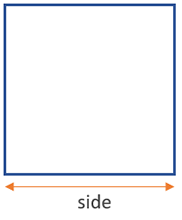This is the simplest figure to calculate as all you need is a single measurement. However, since in most practical situations you need to measure both sides before you know it is a square, it might not be a huge difference, but at least it is easier to calculate.

## Area of a rectangle

The formula for the area of a rectangle is width x height, as seen in the figure below: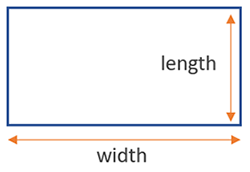You need to take two measurements: the width and the height, and just multiply them together. It is one of the easiest figures to compute an area for. Irregular shapes would often be broken down to a series of rectangles so that their area can be approximately calculated.

## Area of a triangle

The formula for the area of a triangle is height x π x (radius / 2)2, where (radius / 2) is the radius of the base (d = 2 x r), so another way to write it is height x π x radius2. Visual in the figure below: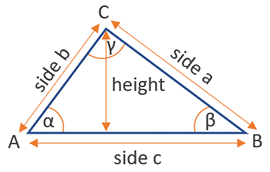Despite the simplicity of the above equation, in specific situations you may not know these two exact measurements. There are multiple rules to calculate a triangle's area: SSS (side-side-side), SAS (two sides and the included angle), SSA (two sides and a non-included angle), ASA (two angles and the included side). For right-angled triangles you can calculate the area by knowing the hypotenuse and the height towards it. All of these are supported by this online area calculator.

## Area of a circle

The formula to find the area of a circle is π x radius2, but the diameter of the circle is d = 2 x r, so another way to write it is π x (diameter / 2)2. Visual on the figure below: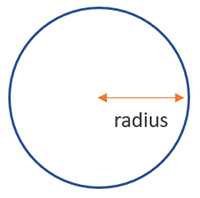For the area of a circle you need just its radius. In most practical situations it would be easier to calculate the diameter instead, which is why our calculator has diameter as an input.

## Area of a parallelogram

The formula for the area of a parallelogram is width x height, as seen in the figure below: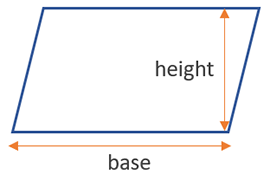Through some simple manipulations it can be shown that a parallelogram can be transformed to rectangle for the purpose of area calculation, thus the formula is basically the same, but we use he height of the parallelogram instead of its side.

## Area of a trapezoid

To find the area of a trapezoid use the equation (base 1 + base 2) / 2 x height. The inputs are as seen in the figure below: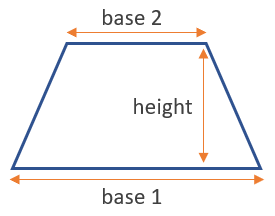This is one of the more complex formulas, but still pretty manageable, especially with the help of an area calculator. A trapezoid can be transformed to a rectangle by knowing the length of each base and its height, so these are the minimum required measurements.

## Area of an ellipse (oval)

The formula for the area of an ellipse is π x major radius x minor radius, as shown on the figure below: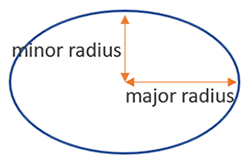The area of an oval is similar to that of a circle, but since it has two radiuses, is a bit different. Here you can measure the two diameters and divide by two to get the two radiuses.

## Area of a sector

The formula for the area of a sector is (angle / 360) x π x radius, but the diameter of the circle is d = 2 x r, so another way to write it is (angle / 360) 2 x π x (diameter / 2). Visual on the figure below: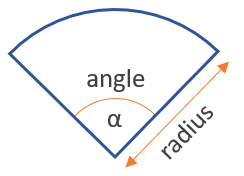Since a sector is just a slice from a circle, the formula is quite similar to that for the area of a circle, with the difference needed to calculate what part of the circle the sector covers. While the radius is easy to measure, the angle is not, unless you have a proper tool at hand, which might be a prerequisite for using our area calculator.

## Area of an octagon

The area of an octagon of regular shape can be found using the formula 2 · (1 + √2) · side2, where a side is as shown below: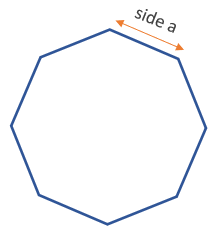It only requires a single measurement, granted that it is already known that the figure is, indeed, a regular octagon. Otherwise one needs to measure all sides first to confirm that they are all equal, before proceeding to calculate its area.

## Where to use an area calculator?

A specialized calculator is obviously handy if you have some geometry homework or if you are allowed to use online tools in class. Other than that, a dedicated calculator might be needed by people involved in certain crafts, engineering, and even in many arts. Many DIY home projects, garden projects, etc. also end up requiring that you calculate one or more areas so you know how much material to purchase, e.g. as in a paint calculator.

#### Cite this calculator & page

If you'd like to cite this online calculator resource and information as provided on the page, you can use the following citation:
Georgiev G.Z., "Area Calculator", [online] Available at: https://www.gigacalculator.com/calculators/area-calculator.php URL [Accessed Date: 28 Sep, 2023].